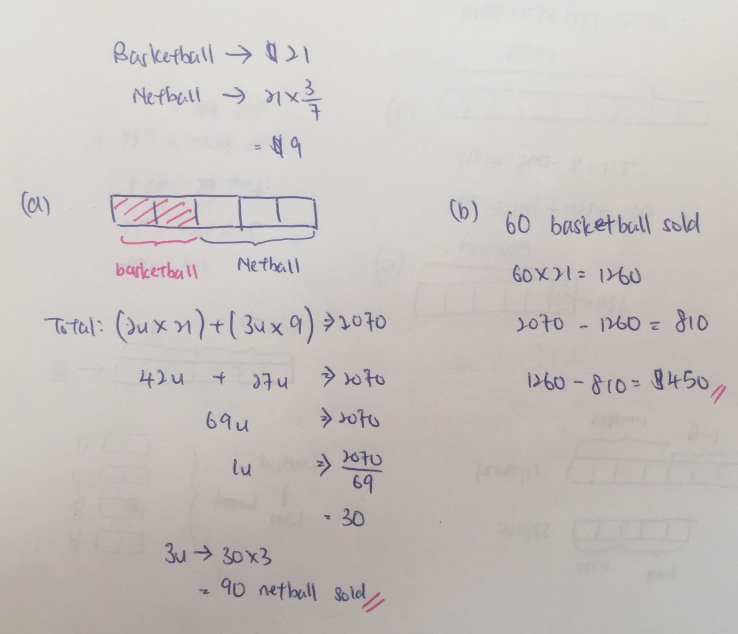# Question

Mrs Tay sold basketballs and netballs. Each basketball cost \$21 each and each netball cost 3/7  as much as the basketball. Mrs Tay sold 1/3 of the balls and collected \$2070. 2/5 of the balls sold were basketballs.

a) How many netballs were sold?

b) How much more money did Mrs Tay receive from the sales of the basketball?

Mrs Tay sold basketballs and netballs. Each basketball cost \$21 each and each netball cost 3/7  as much as the basketball. Mrs Tay sold 1/3 of the balls and collected \$2070. 2/5 of the balls sold were basketballs.

a) How many netballs were sold?

b) How much more money did Mrs Tay receive from the sales of the basketball?

Total balls sold = X

0.4X * 21 + 0.6X * \$9 = \$2070

8.4X + 5.4X = 2070

2070 = 13.8X

X=150 (150 balls sold)

(8.4 x 150) – 5.4 x 150)= \$450

0 Replies 0 Likes0 Replies 0 Likes0 Replies 0 Likes

(a)
21 x (3/7) = 9 (cost of each netball)
basketballs sold : 2 units
2 x 21 = 42
netballs sold : 3 units
3 x 9 = 27
27 + 42 = 69
2070/69 = 30
30 x 3 = 90

(b)
90 x 9 = 810 (total money received form sales of 3 units of netballs)
2070 – 810 = 1260 (total money recfrom sales of 2 units of basketball)
1260 – 810 = 450

Ans : (a) 90 netballs; (b) \$450 more.

1 Reply 0 Likes

Hi

Is there a need to do so?

If there is not, it, 1/3 that is, must be redundant in solving the question.

0 Replies 0 Likes